Register for Maths, Science, English, Reasoning Olympiad Exams Register here | Book Free Demo for Live Olympiad Classes here| Check Olympiad Exam Dates here | Buy Practice Papers for IMO, IOM, HEO, IOEL etc here | Login here to participate in all India free mock test on every Saturday

# Force and Laws of Motion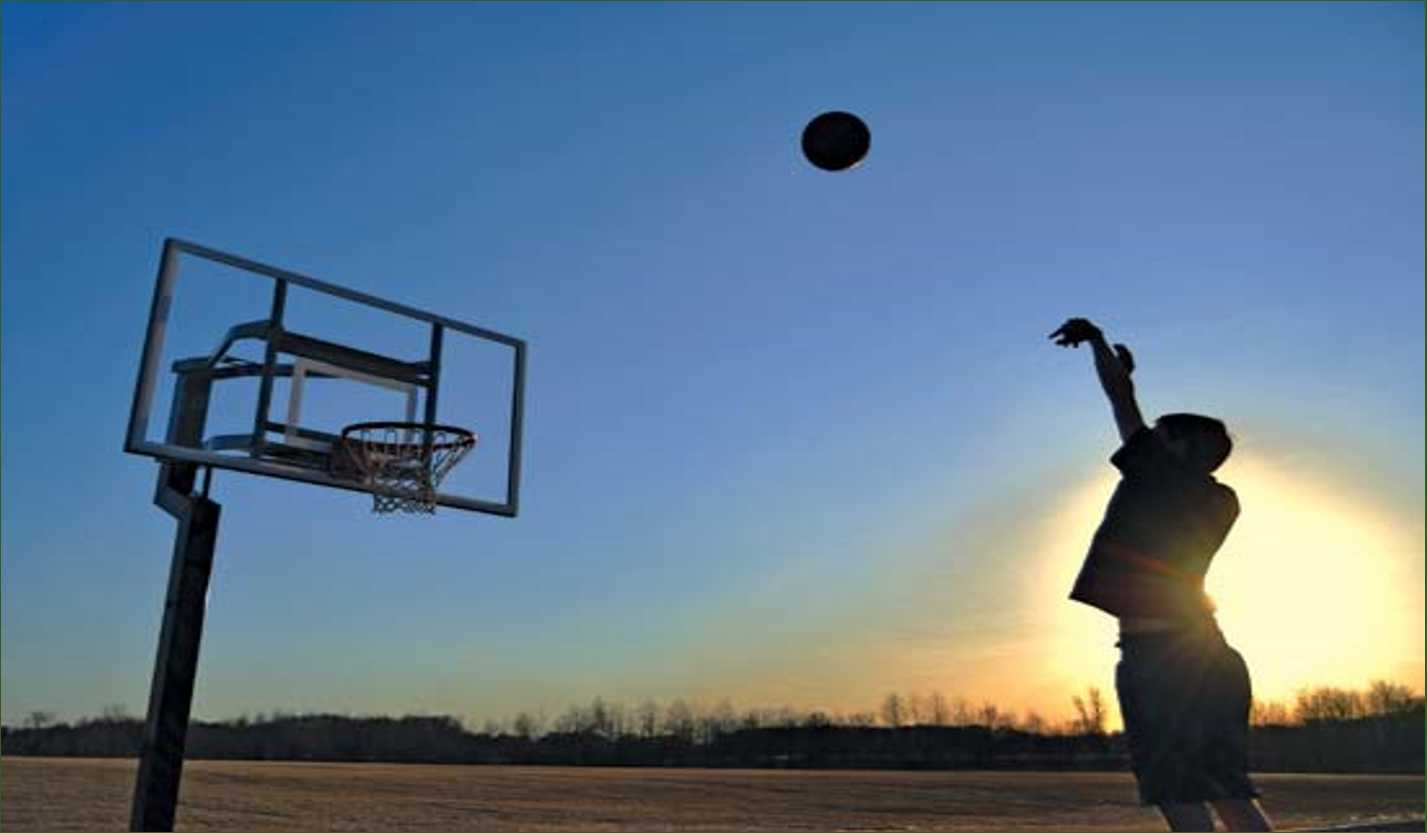For a lot of times, scientists have been researching on how motion actually takes place on planet earth. We had an idea that motion can only be caused by some sort of force on it. But in reality, sometimes even motion takes place without the application of continuous force. Force is generally used to change the velocity of motion or the direction of motion. So Newton’s law of motion and his postulates on the force was a revolution in physics which gave us an idea about how motion takes place and served as the building blocks of the subject mechanics.

Types of Forces:

Balanced Forces: Balance forces are two such forces which act in opposite directions and are equal in size. Whenever there is a balanced force on an object, the object stays still or continues its motion at the same speed and towards the same direction.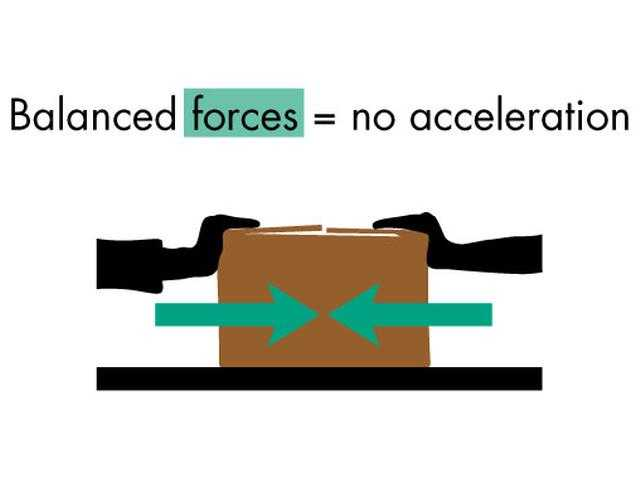Unbalanced Forces: Forces that cause a change in the motion of an object are unbalanced forces. They have a resultant acceleration which causes the motion to move in a certain direction.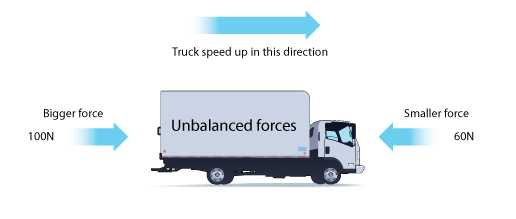First Law of Motion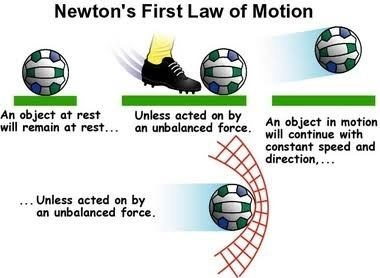Newton's First Law states that an object will remain at rest or in uniform motion in a straight line unless acted upon by an external force. It may be seen as a statement about inertia, that objects will remain in their state of motion unless a force acts to change the motion.

Second Law of Motion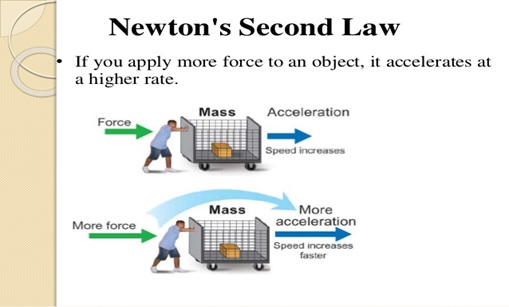Newton's second law of motion can be formally stated as follows: The acceleration of an object as produced by a net force is directly proportional to the magnitude of the net force, in the same direction as the net force, and inversely proportional to the mass of the object.

Third Law of Motion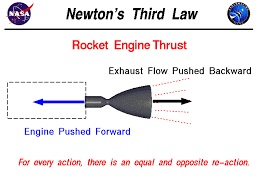For every action, there is an equal and opposite reaction.

Conservation of Matter

All conservation laws such as conservation of momentum, energy, angular momentum, charge etc. are considered to be fundamental laws in physics. These are based on observations and experiments. It is important to remember that a conservation law cannot be proved. It can be verified, or disproved, by experiments. An experiment whose result is in conformity with the law verifies or substantiates the law; it does not prove the law. On the other hand, a single experiment whose result goes against the law is enough to disprove it. The law of conservation of momentum has been deduced from large number of observations and experiments. This law was formulated nearly three centuries ago. It is interesting to note that not a single situation has been realized so far, which contradicts this law. Several experiences of every-day life can be explained on the basis of the law of conservation of momentum.

## Recap

• First law of motion: An object continues to be in a state of rest or of uniform motion along a straight line unless acted upon by an unbalanced force.
• The natural tendency of objects to resist a change in their state of rest or of uniform motion is called inertia.
• The mass of an object is a measure of its inertia. Its SI unit is kilogram (kg).
• Force of friction always opposes the motion of objects.
• Second law of motion: The rate of change of momentum of an object is proportional to the applied unbalanced force in the direction of the force.
• The SI unit of force is kg ms–2. This is also known as Newton and represented by the symbol N. A force of one Newton produces an acceleration of 1 ms–2 on an object of mass 1 kg.
• The momentum of an object is the product of its mass and velocity and has the same direction as that of the velocity. Its SI unit is kg m/s.
• Third law of motion: To every action, there is an equal and opposite reaction and they act on two different bodies.
• In an isolated system (where there is no external force), the total momentum remains conserved.## Quiz for Force and Laws of Motion

 Q.1 Which of them is the correct representation of unit of force Newton? a) Kg.m/s b) Kg.m.s c) Kg.ms-2 d) Kg.m2
 Q.2 1 N force is exerted when _____________. a) When a body moves with a velocity of 1 m/s b) When a body moves with an acceleration of 1 ms-2 c) When a body moves with momentum of 1 kg.m/s d) When 1 kg of mass moves with an acceleration of 1 ms-2
 Q.3 ___________________ the time interval lapsed in change of momentum, ___________________ the force exerted. a) Lesser, Lesser b) More, More c) More, Negative d) Lesser, Larger
 Q.4 The rate of momentum transfer into another object is also termed as _________________. a) Displacement b) Force c) Acceleration d) Velocity
 Q.5 A block of mass 120 g moves with a speed of 6.0 m/s on a frictionless horizontal surface towards another block of mass 180 g kept at rest. They collide and the first block stops. Find the speed of the other block after the collision? a) 2 m/s b) 3 m/s c) 4 m/s d) 5 m/s
 Q.6 A ball of mass 100 g and another ball of 120 g moves towards each other with speeds 6 m/s and 5 m/s respectively. If they stick to each other after colliding, What would be the velocity of the combined mass after the collision? a) 4 m/s b) 1 m/s c) 2 m/s d) 0
 Q.7 A cart of mass 50 kg is moving on a straight track with a speed of 12 m/s. A mass of 10 kg is gently put into the cart. What will be the velocity of the cart after this? a) 60 m/s b) 10 m/s c) 20 m/s d) 30 m/s
 Q.8 A force of 12 N is uniformly distributed over an area of 120 cm 2. Find the pressure in pascals? a) 1000 Pa b) 833.33 Pa c) 10000 Pa d) 100 Pa
 Q.9 Calculate thr force required to be imparted to a car to give it a velocity of 30m/s in 10s. The mass of the car is 1500 kg. a) 1500 N b) 3000 N c) 2500 N d) 4500 N
 Q.10 For how long should a force of 200 N act on a body of 10kg so that it acquires a velocity of 100 m/s? a) 4 s b) 5 s c) 6 s d) 3 s